# Elements of Stochastic Processes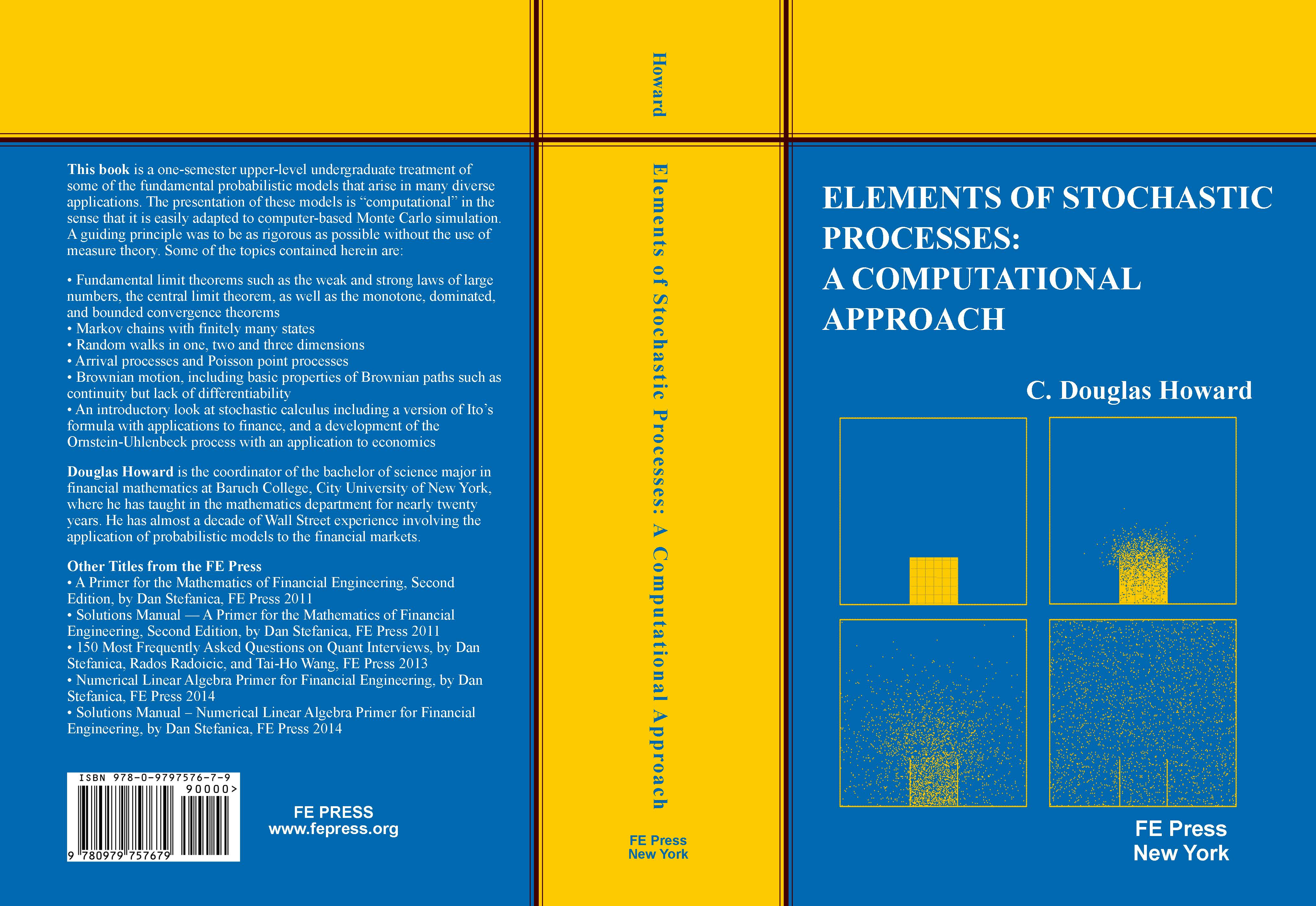Elements of Stochastic Processes: A Computational Approach by Professor C. Douglas Howard, the coordinator of the Financial Mathematics major at Baruch College, City University of New York, and a faculty member in the Baruch MFE Program, was published in November 2017.

This is an advanced undergraduate text on the fundamental probabilistic models that arise in many diverse applications. The presentation of these models is “computational” in the sense that it is easily adapted to computer-based Monte Carlo simulation. A guiding principle was to be rigorous without the use of measure theory.

Elements of Stochastic Processes: A Computational Approach is available for \$44.50, a 10% discount off the list price, for all orders placed before February 15, 2018.

All the books will be signed and personalized by the author.

An Interacting Particle System

Random Walks in Two Dimensions

Itô’s Formula for Brownian Motion

#### FE Press offers discounts of up to 25% off the list price when two or more books are purchased together.

All the books purchased from FE Press are signed and personalized by the authors.

Titles Price ORDER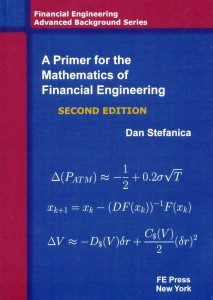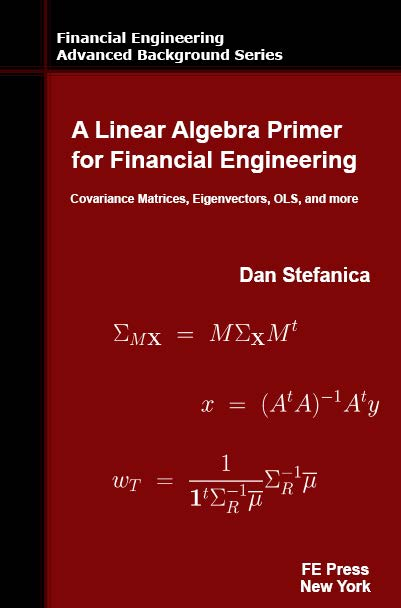• A Primer for the Mathematics of Financial Engineering
• A Linear Algebra Primer for Financial Engineering
• Elements of Stochastic Processes: A Computational Approach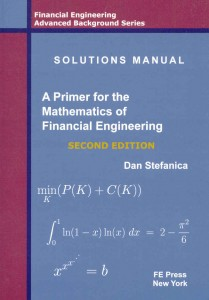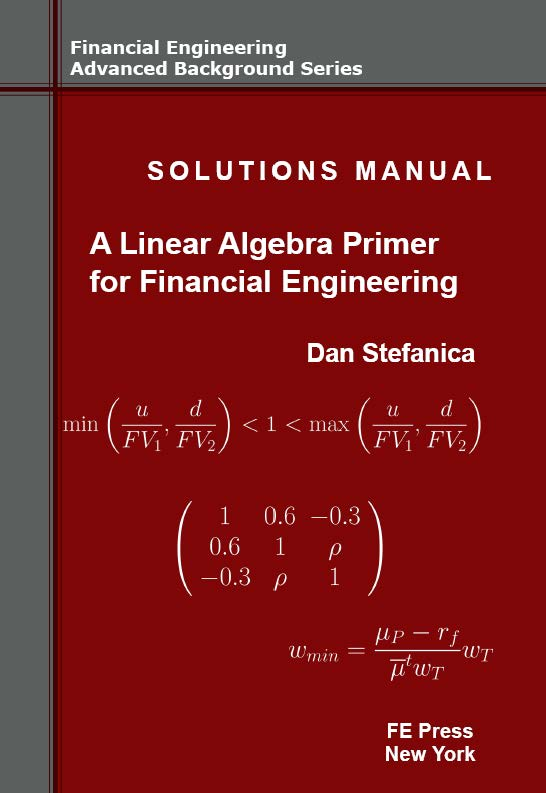• A Primer for the Mathematics of Financial Engineering
• Solutions Manual – A Primer for the Mathematics of Financial Engineering
• A Linear Algebra Primer for Financial Engineering
• Solutions Manual – A Numerical Linear Algebra Primer for Financial Engineering
• Elements of Stochastic Processes: A Computational Approach• A Primer for the Mathematics of Financial Engineering
• Solutions Manual – A Primer for the Mathematics of Financial Engineering
• A Linear Algebra Primer for Financial Engineering
• Solutions Manual – A Numerical Linear Algebra Primer for Financial Engineering
• 150 Most Frequently Asked Questions on Quant Interviews
• Elements of Stochastic Processes: A Computational Approach• A Primer for the Mathematics of Financial Engineering
• Solutions Manual – A Primer for the Mathematics of Financial Engineering
• Elements of Stochastic Processes: A Computational Approach• A Linear Algebra Primer for Financial Engineering
• Solutions Manual – A Numerical Linear Algebra Primer for Financial Engineering
• Elements of Stochastic Processes: A Computational Approach

Topics:

• Fundamental limit theorems such as the weak and strong laws of large numbers, the central limit theorem, as well as the monotone, dominated, and bounded convergence theorems
• Markov chains with finitely many states
• Random walks in one, two and three dimensions
• Arrival processes and Poisson point processes
• Brownian motion, including basic properties of Brownian paths such as continuity but lack of differentiability
• An introductory look at stochastic calculus including a version of Itô’s formula with applications to finance, and a development of the Ornstein-Uhlenbeck process with an application to economics

About the author: Douglas Howard is the coordinator of the bachelor of science major in financial mathematics at Baruch College, City University of New York, where he has taught in the mathematics department for nearly twenty years. He has almost a decade of Wall Street experience involving the application of probabilistic models to the financial markets.

Book Details:

• Softcover: 245 pages
• Publisher: Financial Engineering Press
• List Price: \$49.50
• ISBN-13: 978-0-9797576-7-9